# [从今天开始修炼数据结构]队列、循环队列、PriorityQueue的原理及实现

[从今天开始修炼数据结构]基本概念

[从今天开始修炼数据结构]栈、斐波那契数列、逆波兰四则运算的实现

[从今天开始修炼数据结构]队列、循环队列、PriorityQueue的原理及实现

队列queue是只允许在一端进行插入操作，而在另一端进行删除操作的线性表。

队列是先进先出的线性表，简称FIFO。允许插入的一端称为队尾，允许删除的一端称为队头。如下图所示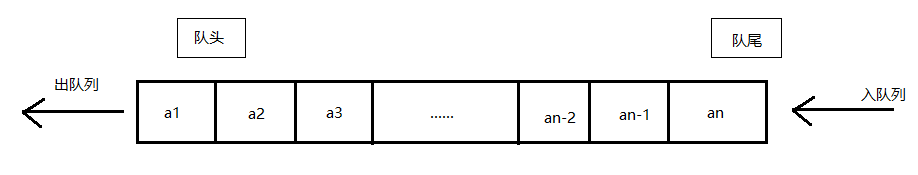例如聊天室消息发送就是队列形式，先发出去的消息会先显示，后发的会后显示。

队列的抽象数据类型与线性表的基本相同，只不过插入删除的位置有限制。

ADT Queue
Data
同线性表，相邻的元素具有前驱后继关系
Operation
InitQueue(*Q):初始化操作
DestroyQueue(*Q):若队列Q存在，销毁他
ClearQueue(*Q):将队列Q清空
QueueEmpty(Q):若队列Q为空，返回true，否则返回false
EnQueue(*Q,e):若Q存在，插入新元素e到队尾
DeQueue(*Q,*e):删除Q中的队首元素，并用e返回其值
QueueLength(Q):返回队列Q的元素个数
endADT

1，在看循环队列之前，我们首先看一下普通的顺序存储队列。

顺序存储队列由数组实现，下标0一端作为初始队首，另一端作为队尾，初始化数组大小应该比要存储的数据要大，以方便插入。我们声明一个队首指针front指向第一个元素，一个队尾指针rear指向队尾元素的下一个位置。

每删除一个元素，队首指针就向后移动一个格子，每插入一个元素，队尾指针也向后移动一个格子。

这样实现的队列有什么弊端？ 由于数组的大小是固定的，尽管设计者可能考虑到声明一个足够大的数组，在经年累月的插入删除后，数组空间总有用完的一天，这时候只能想办法来扩容。但是此时数组的前端还剩下许多由于删除而没有使用的空间，这种现象叫做“假溢出”。为了解决这种现象，我们考虑使用循环队列。

2，循环队列

解决假溢出的办法就是后面满了，就再从头开始，也就是头尾相接的循环。这就是循环队列。当数组后端位置用到最后一个时，让rear指向0位置，这样不会造成指针指向不明的问题。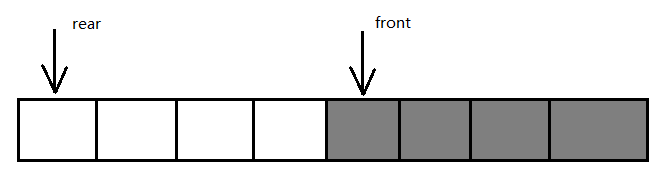现在解决了空间浪费和假溢出的问题，但是现在如何判断队列是满呢？当front=rear时，队列是空还是满呢？

我们设队列的最大长度为QueueSize，且让队列满时，保留一个元素空间。也就是说array.size - 1 = QueueSize。这时队列满的条件为(rear + 1) % QueueSize == front。

另外，通用的队列当前长度计算方法为(rear - front + QueueSize) % QueueSize。

3，循环队列的实现

package Queue;public class ArrayQueueDemo<T> {    /*    仿照JDK1.8 中的 ArrayQueue 实现一个简单的循环队列,有些许不同    JDK 13中为什么没找到ArrayQueue？     */    private Object[] queue;    private int capacity;  //实际要装的数据个数    private int front;    private int rear;    public ArrayQueueDemo(int capacity){        this.capacity = capacity + 1;        this.queue = new Object[capacity + 1];  //+1之后是数组长度，因为要预留一个空位置给rear。 文中提到的QueueSize是没有 + 1 的capacity        this.front = 0;        this.rear = 0;    }    public void add(T data){        queue[rear] = data;        int newRear = (rear + 1) % capacity;        if (newRear == front){            throw new IndexOutOfBoundsException();        }        rear = newRear;    }    public T remove(){        if (isFull()){            throw new IndexOutOfBoundsException();        }        Object removed = queue[front];        queue[front] = null;        front = (front + 1) % capacity;        return (T)removed;    }    /**     * 返回最大队列长度     * @return 最大     */    public int capacity(){        return capacity;    }    /**     * 返回当前队列大小     * @return     */    public int size(){        return (rear - front + capacity - 1) % (capacity - 1);    }    public boolean isFull(){        return (rear + 1) % (capacity - 1) == front;    }}

队列的脸书存储结构，就是线性表的单链表，只不过限制了只能尾进头出。我们把它简称为链队列。为了操作方便，我们把队头指针指向链表的表头，队尾指针指向链表的表尾。空队列时，front和rear都指向头结点。

由于JDK中没有单纯的链式队列，且链式队列的实现很简单，所以我选择在这里实现一下PriorityQueue

优先队列是在入队时自动按照自然顺序或者给定的比较器排序的队列。

优先队列是通过数组表示的小顶堆实现的。小顶堆可以理解为，父结点的权值总是不大于子节点的完全二叉树。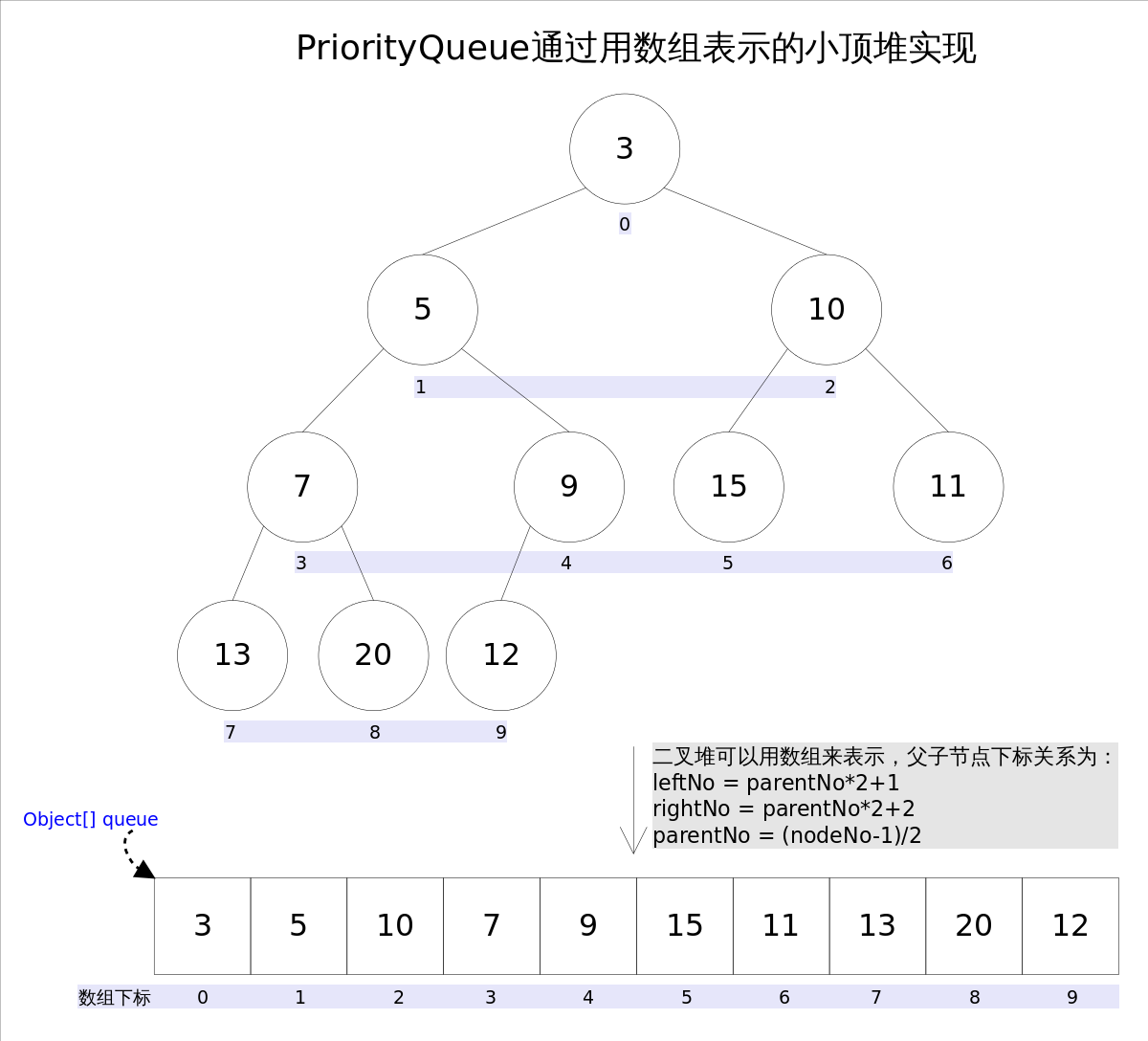leftNo = parentNo*2+1

rightNo = parentNo*2+2

parentNo = (nodeNo-1)/2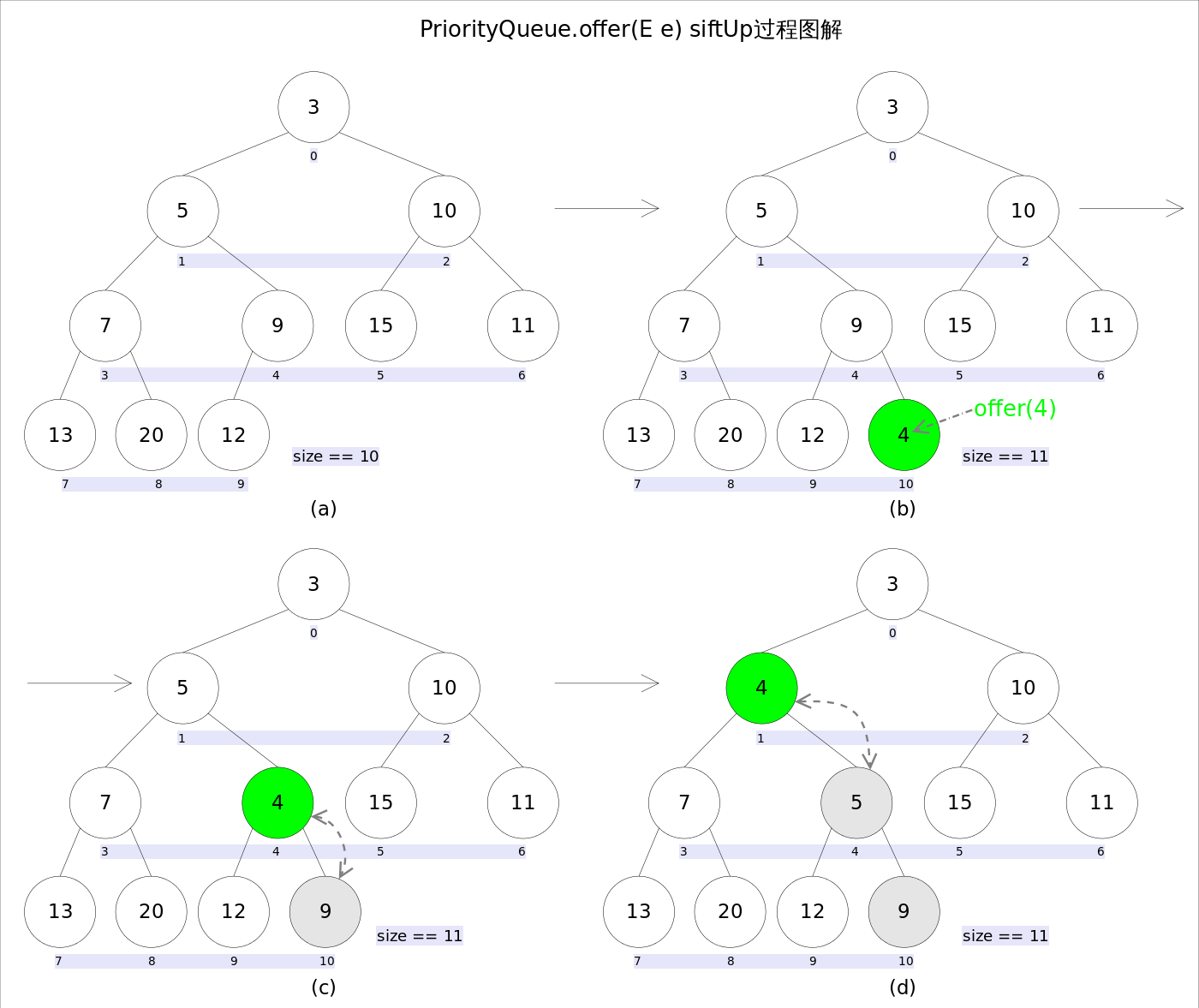Poll()方法的实现原理如下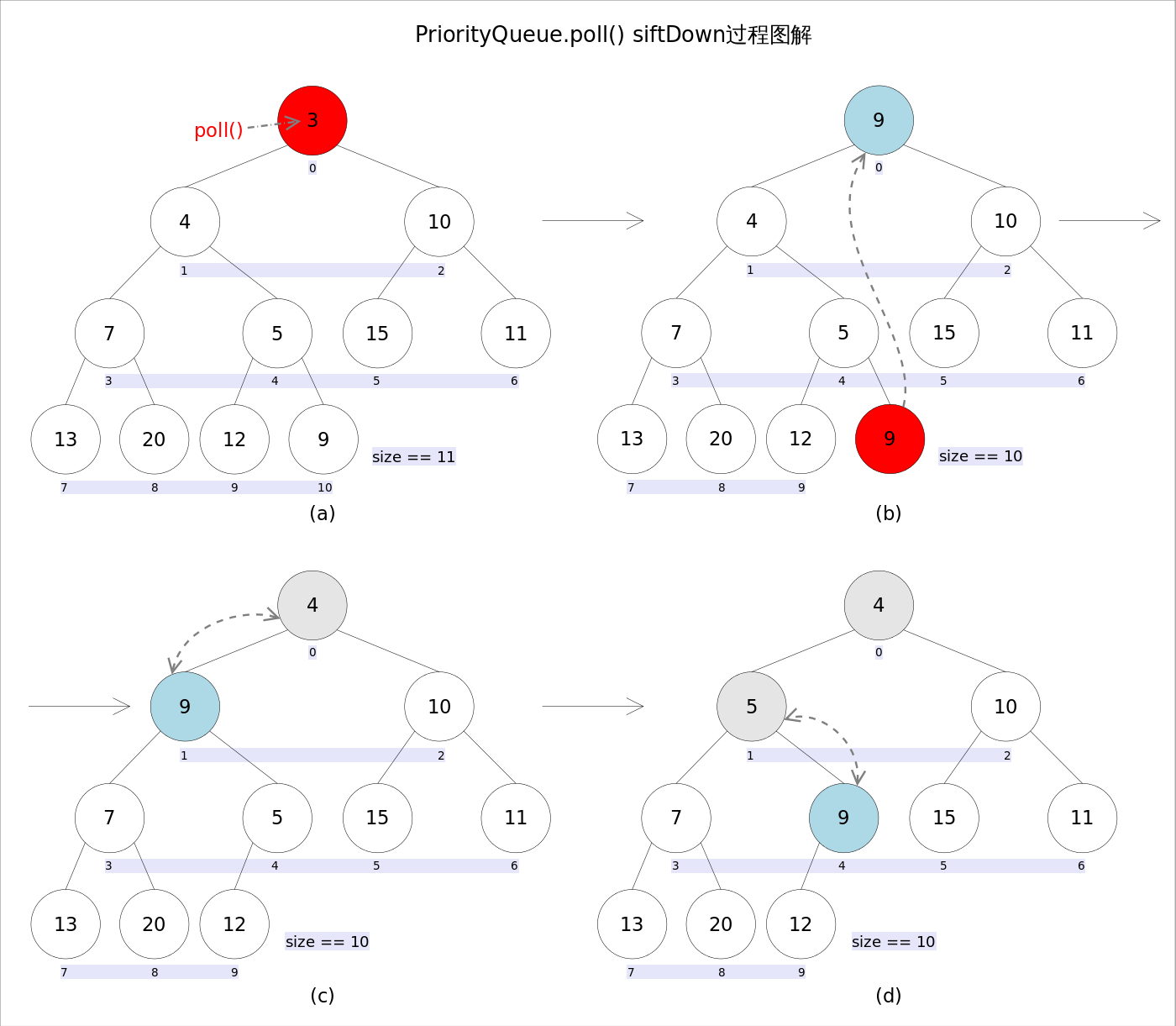PriorityQueue的简易代码实现：

package Queue;

import java.util.Arrays;
import java.util.Comparator;

/*

（Java的优先队列每次取最小元素，C++的优先队列每次取最大元素）。

*/
public class PriorityQueueDemo<T> {
private Object[] queue;
private int size;
private Comparator<? super T> comparator;

public PriorityQueueDemo(int capacity){
queue = new Object[capacity];
size = 0;
}

public PriorityQueueDemo(int capacity,Comparator<? super T> comparator){
this.comparator = comparator;
queue = new Object[capacity];
size = 0;
}

/**
* 这里给出一个堆排序初始化一个小顶堆队列的方法
* @param data 要被排序的数组
* @param comparator 定义比较方式的比较器
*/
public PriorityQueueDemo(T[] data, Comparator<T> comparator) throws Exception {
int capacity = data.length;
queue = new Object[capacity];
size = 0;
this.comparator = comparator;
for (int i = 0; i < data.length; i++) {
}
}

public void add(T data) throws Exception {
int i = size;
if (data == null){
throw new Exception();
}else if (i >= queue.length){
grow(i);
}
if (size == 0){
queue = data;
size++;
}
else {
queue[i] = data;
shiftUp(i, data);
size++;
}
}

public T poll(){
if (size == 0){
throw new NullPointerException();
}
T polled = (T)queue;
int s = --size;
T x = (T)queue[s];
queue = queue[s];
queue[s] = null;
if (s != 0){
shiftDown(0,x);
}

return polled;
}

private void shiftDown(int i, T x) {
//父节点是否大于子节点的标志
//若为true，则需要进入循环。
boolean flag = true;
while(flag) {
int child = (i << 1) + 1;
T smallerChild = (T) queue[child];
int right = child + 1;
if (right >= size || child >= size){
flag = false;
break;
}
if (comparator.compare(smallerChild, (T) queue[right]) > 0) {
child = right;
smallerChild = (T) queue[child];
}
if (comparator.compare(x, smallerChild) <= 0) {
flag = false;
break;
}
queue[i] = smallerChild;
i = child;
}
queue[i] = x;
}

/**
* 新结点上浮
* @param i i的初始值是size的引用,即尾指针的位置。
* @param data 新结点的数据
*/
//理解。
private void shiftUp(int i,T data) {
while(i > 0) {
int parent = (i - 1) >>> 1;
Object e = queue[parent];
if (comparator.compare(data, (T)e) >= 0) {
break;
}
queue[i] = e;
i = parent;
}
queue[i] = data;
}

private void grow(int i) {
Object[] newQueue = Arrays.copyOf(queue, i + 1);
this.size++;
}
}

posted @ 2019-12-02 19:15  Joey777210  阅读(...)  评论(...编辑  收藏